Anzeige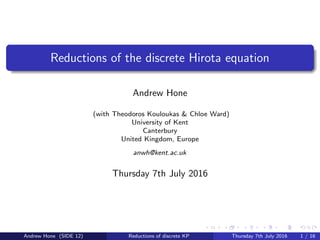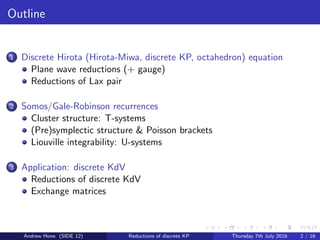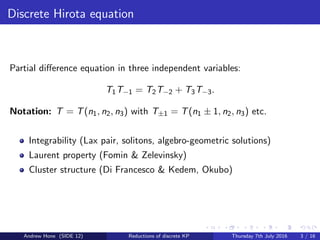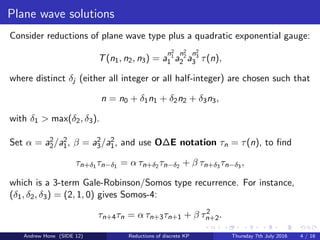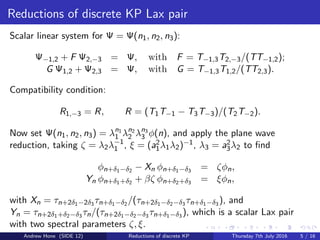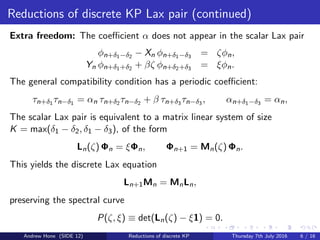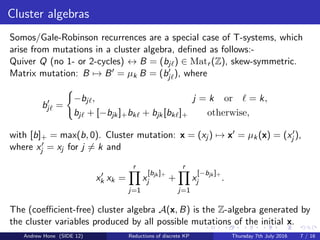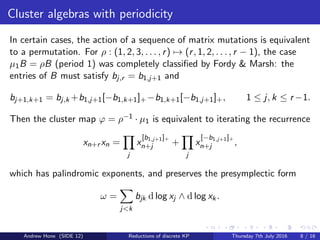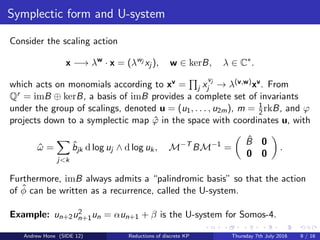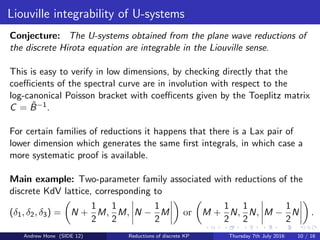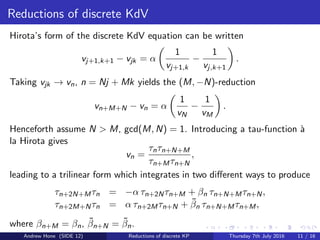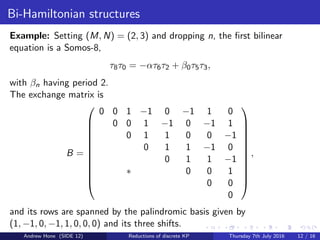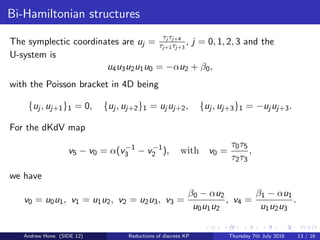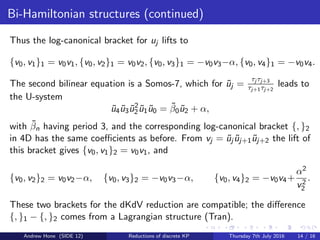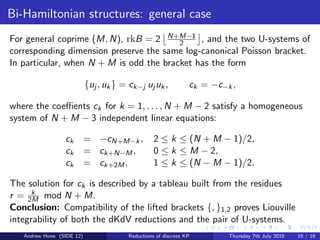1 von 16
Anzeige

### dhirota_hone_corrected

1. Reductions of the discrete Hirota equation Andrew Hone (with Theodoros Kouloukas & Chloe Ward) University of Kent Canterbury United Kingdom, Europe anwh@kent.ac.uk Thursday 7th July 2016 Andrew Hone (SIDE 12) Reductions of discrete KP Thursday 7th July 2016 1 / 16
2. Outline 1 Discrete Hirota (Hirota-Miwa, discrete KP, octahedron) equation Plane wave reductions (+ gauge) Reductions of Lax pair 2 Somos/Gale-Robinson recurrences Cluster structure: T-systems (Pre)symplectic structure & Poisson brackets Liouville integrability: U-systems 3 Application: discrete KdV Reductions of discrete KdV Exchange matrices Andrew Hone (SIDE 12) Reductions of discrete KP Thursday 7th July 2016 2 / 16
3. Discrete Hirota equation Partial diﬀerence equation in three independent variables: T1T−1 = T2T−2 + T3T−3. Notation: T = T(n1, n2, n3) with T±1 = T(n1 ± 1, n2, n3) etc. Integrability (Lax pair, solitons, algebro-geometric solutions) Laurent property (Fomin & Zelevinsky) Cluster structure (Di Francesco & Kedem, Okubo) Andrew Hone (SIDE 12) Reductions of discrete KP Thursday 7th July 2016 3 / 16
4. Plane wave solutions Consider reductions of plane wave type plus a quadratic exponential gauge: T(n1, n2, n3) = a n2 1 1 a n2 2 2 a n2 3 3 τ(n), where distinct δj (either all integer or all half-integer) are chosen such that n = n0 + δ1n1 + δ2n2 + δ3n3, with δ1 > max(δ2, δ3). Set α = a2 2/a2 1, β = a2 3/a2 1, and use O∆E notation τn = τ(n), to ﬁnd τn+δ1 τn−δ1 = α τn+δ2 τn−δ2 + β τn+δ3 τn−δ3 , which is a 3-term Gale-Robinson/Somos type recurrence. For instance, (δ1, δ2, δ3) = (2, 1, 0) gives Somos-4: τn+4τn = α τn+3τn+1 + β τ2 n+2. Andrew Hone (SIDE 12) Reductions of discrete KP Thursday 7th July 2016 4 / 16
5. Reductions of discrete KP Lax pair Scalar linear system for Ψ = Ψ(n1, n2, n3): Ψ−1,2 + F Ψ2,−3 = Ψ, with F = T−1,3T2,−3/(TT−1,2); G Ψ1,2 + Ψ2,3 = Ψ, with G = T−1,3T1,2/(TT2,3). Compatibility condition: R1,−3 = R, R = (T1T−1 − T3T−3)/(T2T−2). Now set Ψ(n1, n2, n3) = λn1 1 λn2 2 λn3 3 φ(n), and apply the plane wave reduction, taking ζ = λ2λ−1 1 , ξ = (a2 1λ1λ2)−1, λ3 = a2 3λ2 to ﬁnd φn+δ1−δ2 − Xn φn+δ1−δ3 = ζφn, Yn φn+δ1+δ2 + βζ φn+δ2+δ3 = ξφn, with Xn = τn+2δ1−2δ3 τn+δ1−δ2 /(τn+2δ1−δ2−δ3 τn+δ1−δ3 ), and Yn = τn+2δ1+δ2−δ3 τn/(τn+2δ1−δ2−δ3 τn+δ1−δ3 ), which is a scalar Lax pair with two spectral parameters ζ, ξ. Andrew Hone (SIDE 12) Reductions of discrete KP Thursday 7th July 2016 5 / 16
6. Reductions of discrete KP Lax pair (continued) Extra freedom: The coeﬃcient α does not appear in the scalar Lax pair φn+δ1−δ2 − Xn φn+δ1−δ3 = ζφn, Yn φn+δ1+δ2 + βζ φn+δ2+δ3 = ξφn. The general compatibility condition has a periodic coeﬃcient: τn+δ1 τn−δ1 = αn τn+δ2 τn−δ2 + β τn+δ3 τn−δ3 , αn+δ1−δ3 = αn, The scalar Lax pair is equivalent to a matrix linear system of size K = max(δ1 − δ2, δ1 − δ3), of the form Ln(ζ) Φn = ξΦn, Φn+1 = Mn(ζ) Φn. This yields the discrete Lax equation Ln+1Mn = MnLn, preserving the spectral curve P(ζ, ξ) ≡ det(Ln(ζ) − ξ1) = 0. Andrew Hone (SIDE 12) Reductions of discrete KP Thursday 7th July 2016 6 / 16
7. Cluster algebras Somos/Gale-Robinson recurrences are a special case of T-systems, which arise from mutations in a cluster algebra, deﬁned as follows:- Quiver Q (no 1- or 2-cycles) ↔ B = (bj ) ∈ Matr (Z), skew-symmetric. Matrix mutation: B → B = µk B = (bj ), where bj = −bj , j = k or = k, bj + [−bjk]+bk + bjk[bk ]+ otherwise, with [b]+ = max(b, 0). Cluster mutation: x = (xj ) → x = µk(x) = (xj ), where xj = xj for j = k and xk xk = r j=1 x [bjk ]+ j + r j=1 x [−bjk ]+ j . The (coeﬃcient-free) cluster algebra A(x, B) is the Z-algebra generated by the cluster variables produced by all possible mutations of the initial x. Andrew Hone (SIDE 12) Reductions of discrete KP Thursday 7th July 2016 7 / 16
8. Cluster algebras with periodicity In certain cases, the action of a sequence of matrix mutations is equivalent to a permutation. For ρ : (1, 2, 3, . . . , r) → (r, 1, 2, . . . , r − 1), the case µ1B = ρB (period 1) was completely classiﬁed by Fordy & Marsh: the entries of B must satisfy bj,r = b1,j+1 and bj+1,k+1 = bj,k +b1,j+1[−b1,k+1]+−b1,k+1[−b1,j+1]+, 1 ≤ j, k ≤ r −1. Then the cluster map ϕ = ρ−1 · µ1 is equivalent to iterating the recurrence xn+r xn = j x [b1,j+1]+ n+j + j x [−b1,j+1]+ n+j , which has palindromic exponents, and preserves the presymplectic form ω = j<k bjk d log xj ∧ d log xk. Andrew Hone (SIDE 12) Reductions of discrete KP Thursday 7th July 2016 8 / 16
9. Symplectic form and U-system Consider the scaling action x −→ λw · x = (λwj xj ), w ∈ kerB, λ ∈ C∗ . which acts on monomials according to xv = j x vj j → λ(v,w)xv. From Qr = imB ⊕ kerB, a basis of imB provides a complete set of invariants under the group of scalings, denoted u = (u1, . . . , u2m), m = 1 2rkB, and ϕ projects down to a symplectic map ˆϕ in the space with coordinates u, with ˆω = j<k ˆbjk d log uj ∧ d log uk, M−T BM−1 = ˆB 0 0 0 . Furthermore, imB always admits a “palindromic basis” so that the action of ˆφ can be written as a recurrence, called the U-system. Example: un+2u2 n+1un = αun+1 + β is the U-system for Somos-4. Andrew Hone (SIDE 12) Reductions of discrete KP Thursday 7th July 2016 9 / 16
10. Liouville integrability of U-systems Conjecture: The U-systems obtained from the plane wave reductions of the discrete Hirota equation are integrable in the Liouville sense. This is easy to verify in low dimensions, by checking directly that the coeﬃcients of the spectral curve are in involution with respect to the log-canonical Poisson bracket with coeﬃcents given by the Toeplitz matrix C = ˆB−1. For certain families of reductions it happens that there is a Lax pair of lower dimension which generates the same ﬁrst integrals, in which case a more systematic proof is available. Main example: Two-parameter family associated with reductions of the discrete KdV lattice, corresponding to (δ1, δ2, δ3) = N + 1 2 M, 1 2 M, N − 1 2 M or M + 1 2 N, 1 2 N, M − 1 2 N . Andrew Hone (SIDE 12) Reductions of discrete KP Thursday 7th July 2016 10 / 16
11. Reductions of discrete KdV Hirota’s form of the discrete KdV equation can be written vj+1,k+1 − vjk = α 1 vj+1,k − 1 vj,k+1 . Taking vjk → vn, n = Nj + Mk yields the (M, −N)-reduction vn+M+N − vn = α 1 vN − 1 vM . Henceforth assume N > M, gcd(M, N) = 1. Introducing a tau-function `a la Hirota gives vn = τnτn+N+M τn+Mτn+N , leading to a trilinear form which integrates in two diﬀerent ways to produce τn+2N+Mτn = −α τn+2Nτn+M + βn τn+N+Mτn+N, τn+2M+Nτn = α τn+2Mτn+N + ˜βn τn+N+Mτn+M, where βn+M = βn, ˜βn+N = ˜βn. Andrew Hone (SIDE 12) Reductions of discrete KP Thursday 7th July 2016 11 / 16
12. Bi-Hamiltonian structures Example: Setting (M, N) = (2, 3) and dropping n, the ﬁrst bilinear equation is a Somos-8, τ8τ0 = −ατ6τ2 + β0τ5τ3, with βn having period 2. The exchange matrix is B =             0 0 1 −1 0 −1 1 0 0 0 1 −1 0 −1 1 0 1 1 0 0 −1 0 1 1 −1 0 0 1 1 −1 ∗ 0 0 1 0 0 0             , and its rows are spanned by the palindromic basis given by (1, −1, 0, −1, 1, 0, 0, 0) and its three shifts. Andrew Hone (SIDE 12) Reductions of discrete KP Thursday 7th July 2016 12 / 16
13. Bi-Hamiltonian structures The symplectic coordinates are uj = τj τj+4 τj+1τj+3 , j = 0, 1, 2, 3 and the U-system is u4u3u2u1u0 = −αu2 + β0, with the Poisson bracket in 4D being {uj , uj+1}1 = 0, {uj , uj+2}1 = uj uj+2, {uj , uj+3}1 = −uj uj+3. For the dKdV map v5 − v0 = α(v−1 3 − v−1 2 ), with v0 = τ0τ5 τ2τ3 , we have v0 = u0u1, v1 = u1u2, v2 = u2u3, v3 = β0 − αu2 u0u1u2 , v4 = β1 − αu1 u1u2u3 . Andrew Hone (SIDE 12) Reductions of discrete KP Thursday 7th July 2016 13 / 16
14. Bi-Hamiltonian structures (continued) Thus the log-canonical bracket for uj lifts to {v0, v1}1 = v0v1, {v0, v2}1 = v0v2, {v0, v3}1 = −v0v3−α, {v0, v4}1 = −v0v4. The second bilinear equation is a Somos-7, which for ˜uj = τj τj+3 τj+1τj+2 leads to the U-system ˜u4 ˜u3 ˜u2 2 ˜u1 ˜u0 = ˜β0 ˜u2 + α, with ˜βn having period 3, and the corresponding log-canonical bracket {, }2 in 4D has the same coeﬃcients as before. From vj = ˜uj ˜uj+1 ˜uj+2 the lift of this bracket gives {v0, v1}2 = v0v1, and {v0, v2}2 = v0v2−α, {v0, v3}2 = −v0v3−α, {v0, v4}2 = −v0v4+ α2 v2 2 . These two brackets for the dKdV reduction are compatible; the diﬀerence {, }1 − {, }2 comes from a Lagrangian structure (Tran). Andrew Hone (SIDE 12) Reductions of discrete KP Thursday 7th July 2016 14 / 16
15. Bi-Hamiltonian structures: general case For general coprime (M, N), rkB = 2 N+M−1 2 , and the two U-systems of corresponding dimension preserve the same log-canonical Poisson bracket. In particular, when N + M is odd the bracket has the form {uj , uk} = ck−j uj uk, ck = −c−k, where the coeﬃents ck for k = 1, . . . , N + M − 2 satisfy a homogeneous system of N + M − 3 independent linear equations: ck = −cN+M−k, 2 ≤ k ≤ (N + M − 1)/2, ck = ck+N−M, 0 ≤ k ≤ M − 2, ck = ck+2M, 1 ≤ k ≤ (N − M − 1)/2. The solution for ck is described by a tableau built from the residues r = k 2M mod N + M. Conclusion: Compatibility of the lifted brackets {, }1,2 proves Liouville integrability of both the dKdV reductions and the pair of U-systems. Andrew Hone (SIDE 12) Reductions of discrete KP Thursday 7th July 2016 15 / 16
16. Merci pour votre attention! Andrew Hone (SIDE 12) Reductions of discrete KP Thursday 7th July 2016 16 / 16
Anzeige